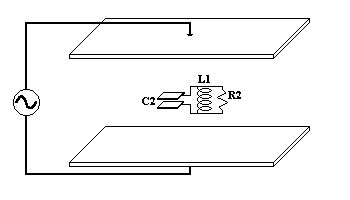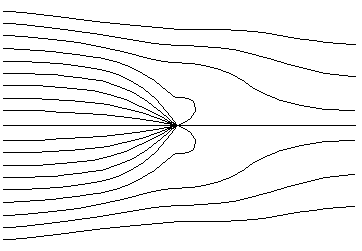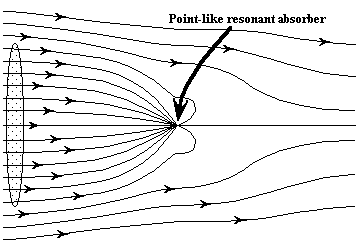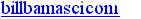TESLA RECEIVE TESLA ARTICLES GOOD STUFF NEW STUFF SEARCH

# Energy Received ByCapacitive "Antennas"1999 W. Beaty BSEE

The ongoing Feb/2000 "energy-sucking antenna" controversy on SCI.PHYSICS.ELECTROMAG (see Feb/2000 thread) , and earlier 1999 on sci.electronics.design prompted me to put together this brief article. It concerns the amount of energy which a tiny, capacitively-coupled antenna can intercept from nearby metal plates.

Since we're having serious disagreements over simple EM physics, first I want to see if everyone can agree on the reality of some basic phenomena which DO NOT involve EM radiation. If we cannot agree on something this simple, then there certainly is no point to fighting over EM travelling waves and any possible "energy sucking" effects involving waves. In addition, if anyone finds a big flaw in the simple stuff below, then that will impact the "energy sucking" idea itself. So first let's fight about simple resonant circuitry! [grin!]

```

```

Here are the abstracts for the physics papers which provoked the whole fight:

1. 1. C. F. Bohren, "How can a particle absorb more than the light incident on it?", Am J Phys, 51 #4, pp323 Apr 1983
2. 2. H. Paul and R. Fischer "Light Absorption by a dipole", SOV. PHYS. USP., 26(10) Oct. 1983 pp 923-926
And here is my first article, the one regarding Nikola Tesla's attempt to broadcast megawatt-levels of EM power between continents using VLF radio: Tesla's Power Receiver

Question: if a capacitively-coupled "antenna" becomes part of an
LC resonator, can it actually receive more energy from an
AC e-field than it otherwise would if the resonator wasn't there?Fig. 1 A capacitive antenna drives a resistor Fig. 2 An inductor is added, forming a tuned circuit

Above we have two variations on a theme: a pair of LARGE parallel metal plates creates a strong AC e-field between themselves, and a pair of SMALL metal plates intercepts a bit of energy from this field. The received energy heats up a load resistor. Assume that all this takes place in the nearfield, where all plate-sizes and distances are far smaller than c/500KHz = 600 meters. Imagine the big plates to be a couple of feet across.

In Fig. 2 on the right, an inductor has been added, and its value is chosen in order to create a tuned circuit with a center frequency the same as the frequency of the voltage-source which drives the large metal plates. In both diagrams the load resistor has been adjusted for maximum received energy. Assume that any stray capacitance of the resistor and inductor are included in other capacitances.

Analysis is very straightforward. Let's add the parasitic capacitances and plug in some real numbers. I'm arbitrarily making the capacitances form a 100:1 voltage divider. If you object, feel free to choose some other ratio.Fig. 3 Comparing the two circuits

First let's choose a value for R1 on the left. Note that the parasitic capacitances "Ca" happen to form a capacitive voltage divider with C1. To adjust R1 for maximum power, we set R1 equal to the Thevinin series reactance created by the Ca-C1 voltage divider, which equals 1/(2*pi*f*100pF) or approximately 3.2K ohms. The voltage which appears across R1 will be .707 times 100V, times the 1:100 voltage division, so V(r1) = 0.7v. Knowing that power = V^2/R, the power received by R1 will be around 160 microwatts. It's fairly small, as you probably expected. After all, the voltage across the C1 plates obviously should be far smaller than the 100V applied to the large plates.

OK, on the right we've added L1, and adjusted it to resonance. Something interesting occurs: the resonant circuit acts like a pure resistor, C2 essentially "vanishes," and the voltage across R2 becomes very large. C2 becomes part of the reactance of the LC circuit, and since I'm assuming lossless components, its reactance is infinite. To adjust R2 for maximum energy, we set it equal to the series reactance of the voltage source, which is two Ca capacitors (2pf) in series, or 1/(2*pi*f*1pf) = 320K ohms. Since C2 and L1 "vanish", the voltage across R2 is simply .707 times the 100v drive, or 71v, and the received power is around 16 milliwatts.

We've found that the maximum power intercepted by each of the two circuits is very different. The circuit with the resonator works better by a factor of 100! By adding a tuned circuit, we've eliminated the 100:1 capacitive voltage divider. And if the large plates were even further apart (but still in the 500KHz nearfield), then the 100x difference between the two circuits would be even greater.

To check my numbers, I divide the power equation for one circuit by the power equation for the other. I find that everything cancels out except the 100:1 from the capacitive voltage divider. The circuit with the resonator receives 100x more power.

Also note that the voltage across C2 is higher than the voltage across C1 by a factor of 100. This implies something interesting. If you held a little bitty electrostatic field meter near C1, it would indicate a low value; a value just about as low as if the C1 plates were not present at all. On the other hand, if you used the same meter to measure the field strength near C2 on the right, you would find it to be very large, almost as if the small plates forming C2 were directly connected to the large, distant driver plates! All this is a consequence of high-Q resonance. Detune the circuit, and the large voltage across C2 goes away.

Pd without tuned circuit: 0.16 mW

Pd with tuned circuit: 16 mW

Increase in e-field near the small plates: 100x

Conclusion: a short dipole antenna doesn't intercept much RF power, but this situation can be amazingly enhanced by adding a resonant circuit. Cool!

OK sci.physics people, all interpretations of the "Energy Sucking" phenomenon aside, do you agree with the following?:

1. Adding a (lossless) tuned circuit can significantly increase the energy throughput capabilities of a small capacitive antenna.
2. The strength of the e-field next to a small capacitive antenna can be much larger when a (lossless) tuned circuit is present.
3. The theory is sound: this effect is real, and is not forbidden by QED, or wave/particle duality, or superposition, or any other well-verified physics principles.
4. If you ACTUALLY BUILT this thing, it would work, although practical considerations might mess things up. The finite value of Q created by real-world coils would reduce the theoretical 100x factor, and it might be hard to keep such sharp tuning from drifting without adding active feedback control.

Feel free to check my calculations. I'm a digital designer and my analog math is rusty.

SIDE NOTE: This is the electrostatic case. The entire article can be rewritten so that the "transmitter" is a large hoop-shaped coil driven by a 500KHz current, and the two receivers are smaller coils nearby, one of which features a tuning capacitor connected across it. Rather than coupled capacitors, we can form loosely- coupled transformers, and the results will be similar. The tuned "antenna coil" will intercept more energy than the RL circuit. And the b-field near the tuned "antenna coil" will be much larger than the b-field near the other.Fig 4. Energy flux lines for the nearfield region of a resonant absorber [from ref#2 above] In figure 4 we see the energy flux (Poynting vector field) surrounding a very small resonant antenna. Plane waves enter from the left, and the antenna occupies a point in the center of the diagram. Clearly the EM energy is being deflected inwards by the dipole field of the small resonator. As the phase of the wave advances with time, so does the phase of the resonator, and the absorbsion occurs during both halves of a 360deg cycle. In this diagram the resonator's dipole pattern is oriented vertically.Fig 5. Circle shows the approximate size of an "absorber disk" which has an area equal to the Effective Aperature (EA) of the resonant antenna. In Fig 5 I've sketched in the profile of an "absorber disk" which has an effective aperture equal to that of the small resonator. Lines of Poynting flux which pass through this disk are deflected so as to strike the tiny resonant absorber. For high-Q absorbers at very long EM wavelengths, the effective aperature can be enormous. In theory a loop antenna a few inches across could gather the same amount of energy as a long-wire antenna hundreds of feet wide. It's just a matter of achiving a high enough "Q" rating.

Suggested by A. Boswell, regarding small-antenna physics:
• Chu, LJ Physical limitations of omni-directional antennas. J. Appl. Phys. 19, 1163- 1175 (1948).
• Hansen, Proc.IEEE Feb. 1981.

```
Created and maintained by Bill Beaty. Mail me at:.